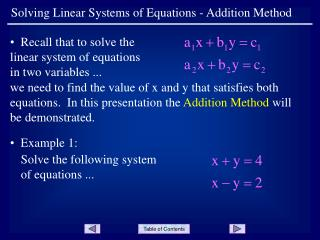DownloadDownload PresentationSolving Linear Systems of Equations - Addition Method

# Solving Linear Systems of Equations - Addition Method

Download Presentation## Solving Linear Systems of Equations - Addition Method

- - - - - - - - - - - - - - - - - - - - - - - - - - - E N D - - - - - - - - - - - - - - - - - - - - - - - - - - -
##### Presentation Transcript

1. Solving Linear Systems of Equations - Addition Method • Recall that to solve the linear system of equations in two variables ... we need to find the value of x and y that satisfies both equations. In this presentation the Addition Method will be demonstrated. • Example 1: Solve the following system of equations ...

2. Solving Linear Systems of Equations - Addition Method • Label the equations as • # 1 and # 2. • Equation # 2 states that x - y has the same value as - 2. Since we can add the same value to both sides of an equation to produce an equivalent equation, we proceed as follows. Slide 2

3. Solving Linear Systems of Equations - Addition Method • Start with equation # 1 ... • Add x - y (the left hand side of equation # 2) to the left hand side ... • Then add - 2 (the right hand side of equation # 2) to the right hand side ... • The result is ... Slide 3

4. Solving Linear Systems of Equations - Addition Method • Solve this equation for x ... • Now use either equation # 1 or equation # 2 to find the value of y. Using equation # 1 ... • The solution to the system is (3, 1), or x = 3 y = 1 Slide 4

5. Solving Linear Systems of Equations - Addition Method • Since equation # 1 was used in the last step, check by substituting the solution values into equation # 2 ... • Notice that in this system the coefficients of the y variables were the same except for sign (+ 1 and - 1). • This is the form that a system must have right before the addition step. When the equations are added, one variable is eliminated, and the result is one equation with one unknown, which is easily solved for. Slide 5

6. Solving Linear Systems of Equations - Addition Method • Example 2: Solve the following system of equations ... • Notice that neither variable meets the condition of coefficients being the same except for sign. This must be accomplished before proceeding. • Multiplying equation # 2 by + 2 yields ... Slide 6

7. Solving Linear Systems of Equations - Addition Method • Now the coefficients on y are the same, except for sign ... • Addition of the equations yields ... Slide 7

8. Solving Linear Systems of Equations - Addition Method • Substitute the value for x into equation # 1 (either equation could be used at this point) ... and solve for y ... • The solution to the system is ... Slide 8

9. Solving Linear Systems of Equations - Addition Method • Example 3: Solve the following system of equations ... • Neither variable meets the condition of coefficients being the same except for sign. The equations can be multiplied by constants to achieve this goal for either variable. • The coefficients of x are 3 and 7. The lcm of 3 and 7 is 21, so multiply each equation by a convenient value to get coefficients of 21, opposite in sign. Slide 9

10. Solving Linear Systems of Equations - Addition Method • Multiply equation # 1 by 7 ... and equation # 2 by - 3 ... • Adding the two equations yields ... Slide 10

11. Solving Linear Systems of Equations - Addition Method • The solution for y is ... • Substituting this value for y in equation # 2 (either equation could be used here) yields ... and the solution is ... Slide 11

12. Solving Linear Systems of Equations - Addition Method • Summary: 1) Multiply the equations by constants so that one of the variables will have the same coefficients, opposite in sign. 2) Add the left sides and then the right sides of the two equations to yield one equation in one variable, for which we can solve. 3) Substitute the given value for the variable into either equation and solve for the other variable. 4) To check the solutions, substitute both values into the equation that was not used in step 3. Slide 12

13. Solving Linear Systems of Equations - Addition Method END OF PRESENTATION Click to rerun the slideshow.